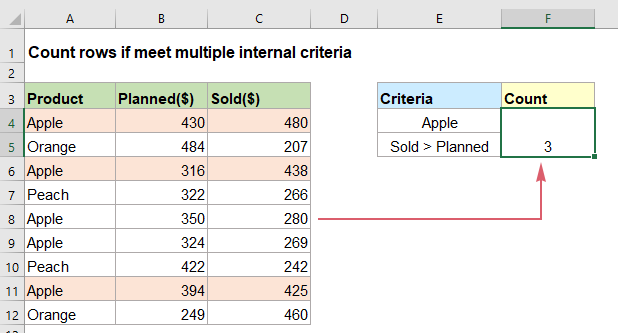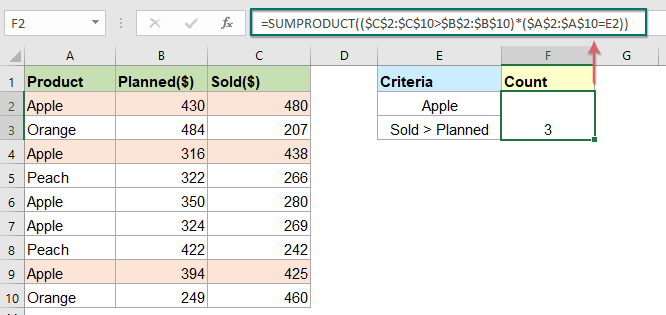## Count rows if meet multiple criteria in Excel

Count the number of rows in a range based on multiple criteria, some of which depends on the logical tests that work at the row-level, the SUMPRODUCT function in Excel may do you a favor.

For example, I have a product report with planned and actual sales, now, I want to count the rows containing the Apple which actual sale is greater than planned sale as below screenshot shown. To solve this task, the most effective function is SUMPRODUCT function.Count rows if meet multiple criteria with SUMPRODUCT function

#### Count rows if meet multiple criteria with SUMPRODUCT function

To count the rows if meet multiple criteria, using the SUMPRODUCT function in Excel, the generic syntax is:

=SUMPRODUCT((logical1)*(logical2))
• logical1, logical2:The logical expressions used to compare the values.

1. For counting the number of Apple rows which actual sale is greater than planned sale, please apply the below formula:

=SUMPRODUCT((\$C\$2:\$C\$10>\$B\$2:\$B\$10)*(\$A\$2:\$A\$10=E2))

Note: In the above formula, C2:C10>B2:B10 is the first logical expression which compares the values in column C to the values in column B; A2:A10=E2 is the second logical expression which checks if the cell E2 exists in column A.

2. Then, press Enter key to get the result you need, see screenshot:##### Explanation of the formula:

=SUMPRODUCT((\$C\$2:\$C\$10>\$B\$2:\$B\$10)*(\$A\$2:\$A\$10=E2))

• \$C\$2:\$C\$10>\$B\$2:\$B\$10: This logical expression is used to compare the values in column C to the values in column B in each row, if the value in column C is greater than value in column B, TRUE is displayed, otherwise, a FALSE will display, and returns the array values as this: {TRUE;FALSE;TRUE;FALSE;FALSE;FALSE;FALSE;TRUE;TRUE}.
• \$A\$2:\$A\$10=E2:This logical expression is used to check if the cell E2 exists in the range A2:A10. So, you will get the result like this: {TRUE;FALSE;TRUE;FALSE;TRUE;TRUE;FALSE;TRUE;FALSE}.
• (\$C\$2:\$C\$10>\$B\$2:\$B\$10)*(\$A\$2:\$A\$10=E2): The multiplication operation is used to multiply these two arrays into one single array to return the result as this: {1;0;1;0;0;0;0;1;0}.
• SUMPRODUCT((\$C\$2:\$C\$10>\$B\$2:\$B\$10)*(\$A\$2:\$A\$10=E2))= SUMPRODUCT({1;0;1;0;0;0;0;1;0}): This SUMPRODUCT adds up the numbers in the array and returns the result: 3.

#### Relative function used:

• SUMPRODUCT:
• The SUMPRODUCT function can be used to multiply two or more columns or arrays together, and then get the sum of products.

#### More articles:

• Count Rows If Meet Internal Criteria
• Supposing, you have a report of product sales of this year and last year, and now, you may need to count products where the sales in this year are greater than last year, or the sales in this year are less than last year as below screenshot shown. Normally, you can add a helper column for calculating the sale difference between the two years, and then use COUNTIF to get result. But, this article, I will introduce the SUMPRODUCT function to get the result directly without any helper column.
• Count Matches Between Two Columns
• For example, I have two lists of data in column A and column C, now, I want to compare the two columns and count if the value in column A found in column C in the same row as below screenshot shown. In this case, the SUMPRODUCT function may be the best function for you to solve this task in Excel.
• Count Number Of Cells Equals To One Of Many Values
• Supposing, I have a list of products in column A, now, I want to get the total number of specific products Apple, Grape and Lemon which listed in range C4:C6 from column A as below screenshot shown. Normally, in Excel, the simple COUNTIF and COUNTIFS functions will not work in this scenario. This article, I will talk about how to solve this job quickly and easily with the combination of SUMPRODUCT and COUNTIF functions.

### The Best Office Productivity Tools

#### Kutools for Excel - Helps You To Stand Out From Crowd

 Popular Features: Find, Highlight or Identify Duplicates  |  Delete Blank Rows  |  Combine Columns or Cells without Losing Data  |  Round without Formula ... Super VLookup: Multiple Criteria  |  Multiple Value  |  Across Multi-Sheets  |  Fuzzy Lookup... Adv. Drop-down List: Easy Drop Down List  |  Dependent Drop Down List  |  Multi-select Drop Down List... Column Manager: Add a Specific Number of Columns  |  Move Columns  |  Toggle Visibility Status of Hidden Columns  |  Compare Columns to Select Same & Different Cells ... Featured Features: Grid Focus  |  Design View  |  Big Formula Bar  |  Workbook & Sheet Manager | Resource Library (Auto Text)  |  Date Picker  |  Combine Worksheets  |  Encrypt/Decrypt Cells  |  Send Emails by List  |  Super Filter  |  Special Filter (filter bold/italic/strikethrough...) ... Top 15 Toolsets:  12 Text Tools (Add Text, Remove Characters ...)  |  50+ Chart Types (Gantt Chart ...)  |  40+ Practical Formulas (Calculate age based on birthday ...)  |  19 Insertion Tools (Insert QR Code, Insert Picture from Path ...)  |  12 Conversion Tools (Numbers to Words, Currency Conversion ...)  |  7 Merge & Split Tools (Advanced Combine Rows, Split Excel Cells ...)  |  ... and more

Kutools for Excel Boasts Over 300 Features, Ensuring That What You Need is Just A Click Away...#### Office Tab - Enable Tabbed Reading and Editing in Microsoft Office (include Excel)

• One second to switch between dozens of open documents!
• Reduce hundreds of mouse clicks for you every day, say goodbye to mouse hand.
• Increases your productivity by 50% when viewing and editing multiple documents.
• Brings Efficient Tabs to Office (include Excel), Just Like Chrome, Edge and Firefox.No ratings yet. Be the first to rate!
This comment was minimized by the moderator on the site
=SUMPRODUCT({Array of True/False}) doesn't count the True values in the array anymore (as of the SUM or COUNT formulaes).
But you can force the convertion of True/False to 1 and 0 by adding the '--' operator right before the array:
=SUMPRODUCT(--{Array of True/False}).
You can also type this operator right after the multiplication sign, giving the strange '*--' operator.

In this exemple, a working formulae would be:
``=SUMPRODUCT(--(\$C\$2:\$C\$10>\$B\$2:\$B\$10)*--(\$A\$2:\$A\$10=E2))``
This comment was minimized by the moderator on the site
Hello Professor X,

You are right in one way. The double negative (--) is one of several ways to coerce TRUE and FALSE values into their numeric equivalents, 1 and 0. Once we have 1s and 0s, we can perform various operations on the arrays with Boolean logic.

But our formula doesn't need the the double negative (--), making the formula more compact. This is because the math operation of multiplication (*) automatically converts the TRUE and FALSE values to 1s and 0s. Have a nice day.

Sincerely,
Mandy
There are no comments posted here yet